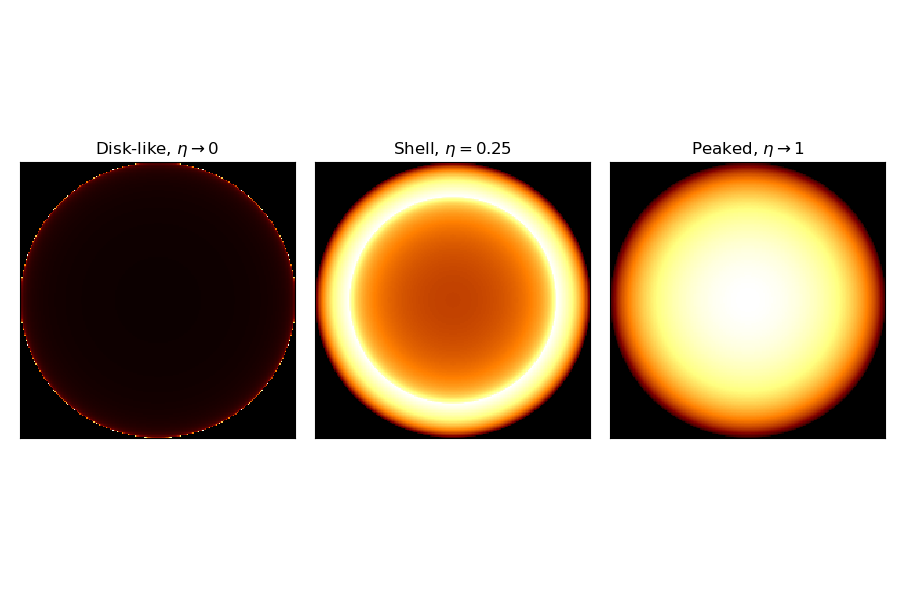# Shell2 spatial model¶

This is a spatial model parametrizing a projected radiating shell.

The shell spatial model is defined by the following equations:

$\begin{split}\phi(lon, lat) = \frac{3}{2 \pi (r_{out}^3 - r_{in}^3)} \cdot \begin{cases} \sqrt{r_{out}^2 - \theta^2} - \sqrt{r_{in}^2 - \theta^2} & \text{for } \theta \lt r_{in} \\ \sqrt{r_{out}^2 - \theta^2} & \text{for } r_{in} \leq \theta \lt r_{out} \\ 0 & \text{for } \theta > r_{out} \end{cases}\end{split}$

where $$\theta$$ is the sky separation, $$r_{\text{out}}$$ is the outer radius and $$r_{\text{in}}$$ is the inner radius.

For Shell2SpatialModel, the radius parameter r_0 correspond to $$r_{\text{out}}$$ and the relative width parameter is given as eta = $$(r_{\text{out}} - r_{\text{in}})/r_{\text{out}}$$ so we have $$r_{\text{in}} = (1-eta) r_{\text{out}}$$.

Note that the normalization is a small angle approximation, although that approximation is still very good even for 10 deg radius shells.

## Example plot¶

Here is an example plot of the shell model for the parametrization using outer radius and relative width. In this case the relative width, eta, acts as a shape parameter.

import matplotlib.pyplot as plt
from gammapy.modeling.models import (
Models,
PowerLawSpectralModel,
Shell2SpatialModel,
SkyModel,
)

tags = [
r"Disk-like, $\eta \rightarrow 0$",
r"Shell, $\eta=0.25$",
r"Peaked, $\eta\rightarrow 1$",
]
eta_range = [0.001, 0.25, 1]
fig, axes = plt.subplots(1, 3, figsize=(9, 6))
for ax, eta, tag in zip(axes, eta_range, tags):
model = Shell2SpatialModel(
lon_0="10 deg",
lat_0="20 deg",
r_0="2 deg",
eta=eta,
frame="galactic",
)
model.plot(ax=ax)
ax.set_title(tag)
ax.set_xticks([])
ax.set_yticks([])
plt.tight_layout()## YAML representation¶

Here is an example YAML file using the model:

pwl = PowerLawSpectralModel()
shell2 = Shell2SpatialModel()

model = SkyModel(spectral_model=pwl, spatial_model=shell2, name="pwl-shell2-model")

models = Models([model])

print(models.to_yaml())


Out:

components:
-   name: pwl-shell2-model
type: SkyModel
spectral:
type: PowerLawSpectralModel
parameters:
-   name: index
value: 2.0
-   name: amplitude
value: 1.0e-12
unit: cm-2 s-1 TeV-1
-   name: reference
value: 1.0
unit: TeV
frozen: true
spatial:
type: Shell2SpatialModel
frame: icrs
parameters:
-   name: lon_0
value: 0.0
unit: deg
-   name: lat_0
value: 0.0
unit: deg
-   name: r_0
value: 1.0
unit: deg
-   name: eta
value: 0.2


Gallery generated by Sphinx-Gallery